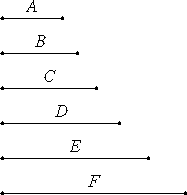# Proposition 9

If as many numbers as we please beginning from a unit are in continued proportion, and the number after the unit is square, then all the rest are also square; and if the number after the unit is cubic, then all the rest are also cubic.

Let there be as many numbers as we please, A, B, C, D, E, and F, beginning from a unit and in continued proportion, and let A, the number after the unit, be square.I say that all the rest are also square.

IX.8

Now it was proved that B, the third from the unit, is square as are all those which leave out one.

I say that all the rest are also square.

VIII.22

Since A, B, and C are in continued proportion, and A is square, therefore C is also square. Again, since B, C, and D are in continued proportion, and B is square, therefore D is also square. Similarly we can prove that all the rest are also square.

Next, let A be a cube.

I say that all the rest are also cubes.

IX.8

Now it was proved that C, the fourth from the unit, is a cube as are all those which leave out two.

I say that all the rest are also cubic.

Since the unit is to A as A is to B, therefore the unit measures A the same number of times as A measures B. But the unit measures A according to the units in it, therefore A also measures B according to the units in itself, therefore A multiplied by itself makes B.

IX.3

And A is cubic. But, if cubic number multiplied by itself makes some number, then the product is also a cube, therefore B is also a cube.

VIII.23

And, since the four numbers A, B, C, and D are in continued proportion, and A is a cube, therefore D also is a cube.

For the same reason E is also a cube, and similarly all the rest are cubes.

Therefore, if as many numbers as we please beginning from a unit are in continued proportion, and the number after the unit is square, then all the rest are also square; and if the number after the unit is cubic, then all the rest are also cubic.

Q.E.D.

## Guide

This proposition says that if a number is a square then all its powers are squares, too. Likewise, the powers of cubes are cubes.

The following theorem is a converse of this one.